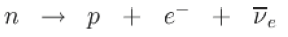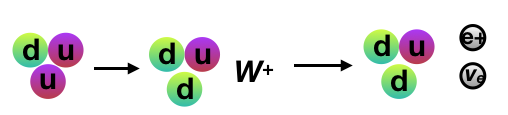### Beta plus and beta minus decay

Beta plus and beta minus decay requires a change in quark character. Repeating the equation for beta minus decay:The weak interaction converts a neutron into a proton while emitting an electron and an anti-neutrino. At the fundamental level (as depicted in the Feynman diagram below), this is due to the conversion of a down quark to an up quark by emission of a Wboson; the Wboson subsequently decays into an electron and an anti-neutrino.

An example of beta emission is carbon 14 decay into nitrogen:

### Quark changes on beta decay

Inside the proton a down quark changes to an up quark which creates a force carrier W- .

travels outside the nucleus  becoming an electron and an electron neutrino. The original neutron has become a proton

### Beta plus decay

In beta plus decay, energy is used to convert a proton into a neutron, a positron and a neutrino:

energy + p  →  +  e  +   νe

So, unlike beta minus decay, beta plus decay cannot occur in isolation because it requires energy input. That is because the mass of the neutron is greater than the mass of the proton. Beta plus decay can only happen inside nuclei when the absolute value of the binding energy of the daughter nucleus is higher than that of the mother nucleus. The difference between these energies goes into the reaction of converting the particles and into the kinetic energy of these particles. Other than that, the sequence is similar - a mirror image of beta minus decay.